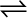7.22 Bromine monochloride, BrCl decomposes into bromine and chlorine and reaches the equilibrium:

2BrCl (g)Br2 (g) + Cl2 (g)

for which Kc= 32 at 500 K. If initially pure BrCl is present at a concentration of
3.3 × 10
–3 mol L–1, what is its molar concentration in the mixture at equilibrium?

Let the amount of bromine and chlorine formed at equilibrium be x. The given reaction is:

Now, we can write,

$\frac{\left[{\mathrm{Br}}_{2}\right]\left[{\mathrm{Cl}}_{2}\right]}{{\left[\mathrm{BrCl}\right]}^{2}}={\mathrm{K}}_{\mathrm{c}}\phantom{\rule{0ex}{0ex}}⇒\frac{\mathrm{x}×\mathrm{x}}{{\left(3.3×{10}^{-3}-2\mathrm{x}\right)}^{2}}=32\phantom{\rule{0ex}{0ex}}⇒\mathrm{x}=18.678×{10}^{-3}-11.32\mathrm{x}\phantom{\rule{0ex}{0ex}}⇒12.32\mathrm{x}=18.678×{10}^{-3}\phantom{\rule{0ex}{0ex}}⇒\mathrm{x}=1.5×{10}^{-3}$

Therefore, at equilibrium,